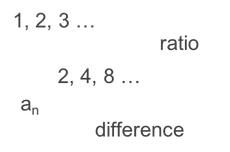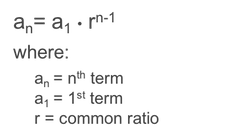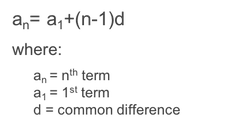# Recursive Formula Calculator

Recursion means “defining a problem in terms of itself.” It is used in writing codes. Its example in math is the Fibonacci sequence(i) = F(i-1) + F(i-2)

What is the recursive formula format?

In the Recursion Method function, call self as a routine procedure several times to perform or solve a problem or task defined. It is used in programming or algorithm writing because it reduces the code of lines.

Recursion is a technique used to solve mathematical or programming problems by dividing them into minor issues and later combining the solutions. It will reduce the problem’s complexity and provide an easy solution.

A recursive formula is a formula that defines each term of a sequence using the preceding term(s). Recursive formulas must always state the initial term, or terms, of the sequence.

## How to use Recursive Function

To determine a recursive Function, we have to follow these steps.

• Find What your function do
• Divide the problem into subproblems
• Develop the solution to your subproblems
• Find the solution to the main problem by combining the subproblem solution
• And Finally, get the answer to the main problem

### How do you find the recursive formula?

an= r × an1

Generally, the recursive function is defined in two parts. It is a statement of the first term along with the formula/ rule related to the successive terms.

## Steps to Use Recursive Formula Calculator

• With the help of this Recursive formula calculator, you can solve the Fibonacci sequence, arithmetic progression, or geometric progression.
• Enter the input value to the relevant fields.
• Enter the value in the if-case statement to check the eligibility and value of the function.
• Add the function of the main problem, like the Fibonacci sequence f(n) = f(n-1)+f(n-2).
• Add the value of n, Where it mentions
• Click on submit button, and boom; you will get the solution to your problems.

## Recursive Sequence Calculator

A recursive sequence, also known as a recurrence sequence, is a sequence of numbers indexed by an integer and generated by solving a recurrence equation.

The formats of recursion sequence is Recursive_sequence(expression; first-term;upper_value;variable)

Example – Recursive_sequence(4*x,-1,3,x);

Where, variable = x, first-term = -1, upper_value = 3, expression = 4*x.

After entering all values, click the submit button, and you will get the output of the geometric sequence using recursion, and you can also plot the series.

In the recursion sequence calculator, Elementary functions are used, like addition, multiplication, Exponentiation, and Binomial coefficients.

• Multiplication – (1+n)a = a+na.
• Exponentiation – a1+n = aan.

Source – Story of Math

MG to ML Calculator

Recursive Formula Calculator – Symbolab / Recursive Sequence Calculator – Symbolab / Recurrence Relation Calculator – Symbolab

How do you calculate an arithmetic sequence?With the help of this formula a_n = a_1 + (n-1)d, where a_1 is the first term of the sequence, a_n is the nth term of the sequence, and d is the common difference

How do you calculate a geometric sequence?With the help of this formula, the nth term of a geometric sequence is a_n = a_1 * r^(n-1), where a_1 is the first term of the sequence, a_n is the nth term of the sequence, and r is the common ratio.

How do you calculate a Fibonacci sequence?

The formula for the nth term of a Fibonacci sequence is a_n = a_(n-1) + a_(n-2), where a_1 = 1 and a_2 = 1.

What are the 3 types of sequences?

The most typical types of sequences are arithmetic, geometric, and Fibonacci.

What is an Arithmetic Sequence?An arithmetic sequence is a number sequence in which the term is gained by adding a fixed number to the last term. Formula is a_n = a_1 + (n-1)d, where a_1 means first term, a_n is nth term and d is common difference

What is a geometric Sequence?

A geometric sequence is a number sequence in which the term is gained by multiplying a fixed number by the last term. The formula is a_n = a_1 * r^(n-1), where a_1 means the first term, a_n is the nth term, and r is the common ratio obtained by dividing the current term by the previous term.

What is a Fibonacci Sequence?

A Fibonacci sequence is a number sequence in which the term is the sum of the previous two terms. The formula is a_n = a_(n-1) + a_(n-2), where a_1 = 1 and a_2 = 1. The sequence is the sum of the previous two terms.